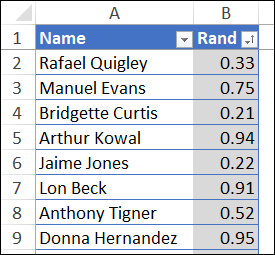## Choose Random Names From Excel List – Formulas No Macros

Do you ever need to choose random names from a list in Excel? Maybe your charity is picking winners for a raffle, or your blog is doing a giveaway, or you need “volunteers” for a special project at work. As long as you have a list of the names, or ticket numbers, or anything else, Excel can do a random draw for you.

### Choose Random Names in Excel

There’s a new sample file on my Contextures site, and you can use it to randomly choose items from a list. The workbook uses formulas to do the choosing – there aren’t any macros to worry about.

There are 2 “Chooser” sheets in the workbook:

1. Chooser365 – For Excel 365 – Spill functions in cells D3 and E3 list numbers and names
2. ChooserALL – For all Excel versions – INDEX/MATCH functions, limit of 10 names

Which version will you use? Do you have Excel 365 now? Or are you still using an older version of Excel?

### Name List

In the Name Chooser workbook, there a list of names, formatted as an Excel table. The table has 2 columns:

• Names – Type names (or whatever)  in this column,. You can sort the names, or leave them as is
• Rand – Has a simple RAND formula to create a random number: =RAND()

Both columns in the table have been named, and the names are used in formulas on the Chooser sheets

• NameList
• RandList### How Many Names to Choose?

On each Chooser sheet, there’s a cell where you type the number of names that you want to randomly choose.

Here’s the Chooser365 sheet, where we’d like to see 3 randomly-selected names.

• Or maybe they were selected to work the weekend shift!### Choose a Scary Movie

In the sample file, there’s a list of fake names, but you could put any list of unique items there instead.

For example, it’s almost Halloween, so put all your favourite scary movie titles there. Then, type a number, and watch those movies on Netflix this weekend!### Excel 365 Formulas

In the Excel 365 version of the Chooser, Excel’s new spill functions are used. There are only 2 formulas on the worksheet, and the results spill down, into as many rows as needed.

The SEQUENCE formula in cell D3 creates a list of numbers for the names, based on the number in cell B3.

• =IFERROR(SEQUENCE(B3),”–“)

And this formula in cell E3 creates the random list of names, using the new FILTER function to pull the list.

• =IFERROR(FILTER(NameList,RandList>=LARGE(RandList,B3)),”–“)

### How It Works

Here’s how the Excel 365 random name formula works:

• First, the LARGE function finds the nth largest number in the RandList range, based on the number in cell B3.
• In this example, that number is 3, so it will return the 3rd largest number in the RandList range.
• Next, the FILTER function returns names from the NameList range, where the Rand number is greater than or equal to the nth largest Rand number.
• Finally, if something causes an error, such as no number entered in B3, the IFERROR function shows two hyphens as the result.

### Save the Selected Names

IMPORTANT: Every time the worksheet calculates, the list of randomly-chosen names will update automatically.

If you want to save the list of names that were selected, copy the names, and paste them as values, somewhere else.

### Excel Formulas – ALL Versions

The other Chooser sheet has formulas that can be used in all versions of Excel. The results don’t spill down the sheet, so I set up 10 rows with formulas, to show the randomly selected names.

### Number List

This formula is entered in cell D3, and copied down to cell D12. These formulas create a list of numbers for the names, based on the number in cell B3.

• =IF(MAX(D\$2:D2)=\$B\$3,””,SUM(D2,1))

### Name List

This formula, entered in cell E3 and copied down to E12, creates the random list of names.

• =IF(D3=””,””,INDEX(NameList, MATCH(LARGE(RandList,D3), RandList,0)))

### How It Works

Here’s how the Excel All Versions random name formula works:

• First, the LARGE function finds the nth largest number in the RandList range, based on the number in column D, in each row.
• In cell E3, that would be the largest Rand number, and in E4, it’s the 2nd largest, and so on
• Next, the MATCH function returns a row number, within the RandList range, where that Rand number is found.
• Then, the INDEX function returns the name from that row number within the NameList range
• Finally, if something causes an error, such as no number entered in B3, the IFERROR function shows two hyphens as the result.

See more INDEX function and MATCH Function examples on my Contextures site.### Get the Random Name Chooser Workbook

To get the random name chooser workbook, and more details on how it’s set up, go to the RAND and RANDBETWEEN page on my Contextures site.

The formulas on the Chooser365 sheet work in Excel 365, or other versions with the new spill functions. For other versions of Excel, use the ChooserALL sheet. The zipped file is in xlsx format, with no macros.

____________________________

### Choose Random Names From Excel List – Formulas No Macros____________________________